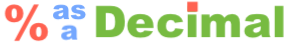24.01 as a DecimalWhen you ask, "What is 24.01 as a decimal?", we assume you want to know what 24.01 percent is as a decimal. In other words, 24.01 percent converted to decimal.

First, we will tell you why it is useful to know 24.01 as a decimal.

Normally, to calculate 24.01 percent, you would multiply a number by 24.01 percent and then you would take the product of that and divide it by 100 to get the answer.

Instead, you can simply multiply a number by 24.01 as a decimal to get the answer.

24.01 percent means 24.01 per hundred. Therefore, to get 24.01 as a decimal, all you have to do is divide 24.01 by 100 like so:

24.01 ÷ 100 = 0.2401

Shortcut: When you divide anything by 100, just move the decimal point two places to the left.

Percent as a Decimal
Need another percent as a decimal? Convert another percent to decimal below:

24.02 as a Decimal
Here is the next percent in our How-to Catalog that we converted to a decimal.

Remember This:
24.01 as a decimal is 0.2401 and you can multiply 0.2401 by a number to get 24.01 percent of that number.